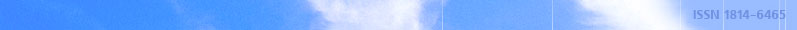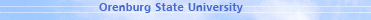december 07, 2021 RU/EN
 Headings of Vestnik Pedagogics Psychology Other

 Search Author Article

# 2006, № 9

Frolov S.S., Shevelenko V.D., Gusarov A.A. APPROXIMATION METHOD OF SINUSOIDAL EQUAL-AMPLITUDE POLYNOMIALOne of approximation methods of equal-amplitude trigonometrical polynomials having equal permanent discrete spectrum, - approximation method of sinusoidal polynomial is suggested in this article. Analytical expressions for discrete harmonic spectrum of approximate function are given and its determinant characteristics – coefficient of parasite spectrum harmonics and unevenness of harmonics amplitudes at working sphere of spectrum are calculated in this work.Editor-in-chief Sergey Aleksandrovich MIROSHNIKOV